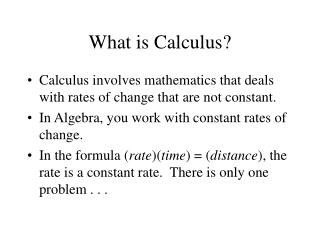DownloadDownload PresentationWhat is Calculus?

# What is Calculus?

Download Presentation## What is Calculus?

- - - - - - - - - - - - - - - - - - - - - - - - - - - E N D - - - - - - - - - - - - - - - - - - - - - - - - - - -
##### Presentation Transcript

1. What is Calculus? • Calculus involves mathematics that deals with rates of change that are not constant. • In Algebra, you work with constant rates of change. • In the formula (rate)(time) = (distance), the rate is a constant rate. There is only one problem . . .

2. Here is a case of a sharp decrease in velocity.

3. Here, this bungee jumper experiences acceleration, followed by deceleration

4. Differential Equations: The Study of Rates of Change • In the study of differential equations, we are able to take a rate equation and “solve it”. • To “solve” a differential equation means to write the equation in the form that does not contain rates. • Have you ever heard of “exponential growth”?

5. Exponential Growth • Exponential Growth occurs when the rate of growth of some “thing”is directly proportional to the amount of that “thing” present. • Example: Plants in a garden grow exponentially. • A possible equation describing this growth could be • dy/dt = 0.10y where y = the mass of the plant after “t” days and the plant increases in mass approximately 10% (0.10) each day. • What is dy/dt?

6. What is dy/dt? • dy/dt is the “Rate of Growth” of the plant measured in mass units per time. • For example if y = 20grams, dy/dt = 0.1(20) = 2 grams of new growth per day. But the next day, the mass of the plant is about 22 grams so, dy/dt = 0.1(22) = 2.2 grams of growth and so on . . . • The growth rate keeps growing! What is the solution?

7. The Solution • Using “techniques” from Calculus, we may “solve” the differential equation dy/dt=0.10y to get the equation • y = 10e0.095t where we use y=10 for day 0. • A “picture” is worth a lot here!

8. Mass of a Garden PlantGraph of y = 10e0.095t

9. How Bad Shocks Affects Car Ride Quality • We can use Calculus and Differential Equations to actually simulate the ride of a car with bad shock absorbers! • In the suspension system of a car, there are two major components: 1) Springs to cushion the ride. 2) Shocks to “dampen” the bounce.

10. Vertical Acceleration in a Car Ride When Hitting a Bump • When you hit a bump while driving a car, there is a lot of “up and down” change in position and acceleration and deceleration occurring. We use dy/dt to represent the change in position with respect to time and we use d2y/dt2 to represent acceleration.

11. Equation For This System • The second order differential equation is m* d2y/dt2 + kd*dy/dt + ks*y = 0 where m = mass of rear end of vehicle kd = the damping coefficient due to the shocks ks = the spring coefficient • Note that “damping” is primarily achieved by the shock absorber but additional damping occurs due to frictional heat losses. • After looking up a value for “m” and experimentally determining kd and ks, the equation obtained is 24.2*d2y/dt2 + 400*dy/dt + 2400*y = 0

12. Comparisonof Solutions Graphed Good Shocks 24.2*d2y/dt2 + 400*dy/dt + 2400*y = 0 Bad Shocks 24.2*d2y/dt2 + 200*dy/dt + 2400*y = 0 Very Bad Shocks 24.2*d2y/dt2 + 100*dy/dt + 2400*y = 0 Note: For this problem, the “solutions” are obtained “graphically”.

13. 1986 Toyota CelicaSuspension System Rear Shocks in Good Condition Vertical Displacement of Rear in Feet is Plotted Against Time in Seconds

14. 0.25 Seconds

15. 0.50 Seconds

16. 0.75 Seconds

17. 1.00 Seconds

18. 1.25 Seconds

19. 1986 Toyota CelicaSuspension System Rear Shocks in BAD Condition Viscosity is 1/2 as Much Vertical Displacement of Rear in Feet is Plotted Against Time in Seconds

20. 0.25 Seconds

21. 0.50 Seconds

22. 0.75 Seconds

23. 1.00 Seconds

24. 1.25 Seconds

25. 1986 Toyota CelicaSuspension System Rear Shocks in VERY BAD Condition Viscosity is 1/4 as Much Vertical Displacement of Rear in Feet is Plotted Against Time in Seconds

26. 0.2 Seconds

27. 0.4 Seconds

28. 0.6 Seconds

29. 0.8 Seconds

30. 1.0 Seconds

31. 1.2 Seconds

32. 1.4 Seconds

33. 1.6 Seconds

34. 1.8 Seconds

35. 2.0 Seconds

36. 2.2 Seconds